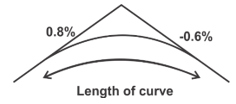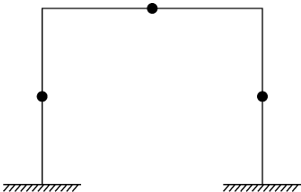Engineering Jobs   »   DFCCIL Civil engineering quiz

# DFCCIL’21 CE: Weekly Practices Quiz. 08-Aug-2021

Quiz: Civil Engineering
Exam: DFCCIL
Topic: Miscellaneous

Each question carries 1 mark
Negative marking: 1/4 mark
Time: 12 Minutes

Q1. The maximum depth of neutral axis in a RC beam for Fe 415 grade of steel is:
(a) 0.53d
(b) 0.46d
(c) 0.67d
(d) 0.48d

Q2. The slope correction for a length of 30 m along a gradient of 1 in 20 is ______.
(a) 3.75 cm
(b) 0.375 cm
(c) 37.5 cm
(d) 2.75 cm

Q3. Degree of static indeterminacy of a rigid-jointed plane frame having 15 members, 3 reactions components and 14 joints is
(a) 8
(b) 6
(c) 5
(d) 3

Q4. If all the dimensions of a prismatic bar of square cross-section suspended freely from the ceiling of a roof are doubled then the total elongation produced by its own weight will increase.
(a) Eight times
(b) Four times
(c) Three times
(d) Two times

Q5. If the volume of a liquid weighing 3000 kg is 4 cubic meters, 0.75 is its
(a) Specific weight
(a) Specific mass
(c) Specific gravity
(d) None of these

Q6. A dry soil has mass specific gravity of 1.35. If the specific gravity of solids is 2.7 then the void ratio will be
(a) 0.5
(b) 1.0
(c) 1.5
(d) 2.0

Q7. The thermal expansion coefficient (α) of steel is:
(a) 13×10^(-6/°C) and closely resembles to α of concrete.
(b) 11×10^(-6/°C) and differs widely from α of concrete
(c) 12×10^(-6/°C) and close to α of concrete.
(d) 14×10^(-6/°C) but nearly equal to α of concrete

Q8. In some brick masonry walls, patches of whitish crystal were found on the exposed surfaces, also chipping and spalling of bricks took places from the same walls. Which among the following are the causes of these defects?
1. Settlement of foundation
3. Sulphate attack
4. Efflorescence
(a) 1 and 2
(b) 2 and 3
(c) 2 and 4
(d) 3 and 4

Q9. The length of a curve connecting two uniform gradients of +0.8% and -0.6%, the rate of change of grade being 0.1% per 30 m, will be
(a) 220 m
(b) 320 m
(c) 420 m
(d) 520 m

Q10. Which one of the following statements is correct? An indeterminate building frame may be converted to a determinate one by assuming
(a) hinges at mid-height of columns
(b) hinges at mid-span of the beams
(c) hinges at both mid-height of columns and midspan of beams
(d) one support as fixed at base and other support on rollers

Q11. A timber beam of effective span ‘l’ and of cross-section b × d is said to be laterally supported if d/b and l/b are respectively
(a) Less than 1 and less than 48
(b) Less than 2 and less than 49
(c) less than 3 and less than 50
(d) Less than 4 and less than 51

Q12. The shape factor for a diamond section is
(a) 1.00
(b) 2.00
(c) 1.50
(d) 2.50

Solutions

S1. Ans.(d)
Sol.

 Grade of steel Depth of neutral axis () Fe 250 0.53d Fe 415 0.48d Fe 500 0.46d

S2. Ans.(a)
Sol. Given, Length=30m, h= 1/(√(〖(20)〗^2+1) )×30=1.5m.
Correction for a slope = h^2/2l = 〖1.5〗^2/(2×30) = 0.0375m. =3.75 cm.

S3. Ans.(b)
Sol. Given, member (m) = 15
Reaction (r_e) = 3
Joints (j) = 14
D_S= ?
D_s=(3m+r_e )-3j
=(3×15)+3-(3×14)
=48-42
=6

S4. Ans.(b)
Sol. Total elongation in a prismatic bar due to its self-weight is given by-
▭(∆L=(yL^2)/2E)
Now, length of bar is doubles then Total elongation in bar will be-
∆L^’=(γ×(2L)^2)/2E
=4×(γL^2)/2E
▭(∆L^’=4∆L)

S5. Ans.(c)
Sol. Given,
Weight of liquid (W) = 3000 kg
Volume of liquid (V) = 4 m³
Density of liquid (ρ) =W/V=3000/4 = 750 kg/m³
Specific gravity (G) = (Density of liquid (ρ))/(Density of water(ρ_w ) )
=750/1000
=0.75

S6. Ans. (b)
Sol. Given,
G_m=1.35
G_s=2.7
e= ?
G_m=G_s/(1+e)
1.35=2.7/(1+e)
▭(e= 1.0)

S7. Ans.(c)
Sol. Coefficient of thermal expansion (α) for steel is 12×10^(-6)/°c
Coefficient of thermal expansion for Concrete is 6×10^(-6)/°c to 12×10^(-6)/°c

S8. Ans.(d)
Sol.
The patches of whitish crystal were found on the exposed surface is due to efflorescence.
the chipping and spalling of bricks are due to sulphate attack.

S9. Ans.(c)
Sol.Length of curve =(0.8-(-0.6))/0.1 ×30
▭(L=420m.)

S10. Ans.(c)
Sol. An indeterminate building frame is statically indeterminate to third degree hence to make it determinate we assume hinges at both mid – height of column and midspan of beams.S11. Ans.(c)
Sol. AS per IS 883:1994, the minimum width of beam or any flexural member shall not be less than l/50 or 50 mm of the span whichever is greater. The depth of beam of any flexural member shall not be taken more than 3 times of its width.

S12. Ans.(b)
Sol. The shape factor for a diamond section is 2.00.

Sharing is caring!